# Texas Go Math Grade 1 Lesson 11.2 Answer Key Model Subtraction

Refer to our Texas Go Math Grade 1 Answer Key Pdf to score good marks in the exams. Test yourself by practicing the problems from Texas Go Math Grade 1 Lesson 11.2 Answer Key Model Addition.

## Texas Go Math Grade 1 Lesson 11.2 Answer Key Model Subtraction

Unlock the Problem

Tom has 6 crayons in a box.
He takes 2 crayons out of the box.
How many crayons are in the box now? lip How can you use a model to find out?

What information am I given?
___________ crayons in a box
___________ crayons taken out
Tom has 6 crayons in a box.
He takes 2 crayons out of the box.

Plan

What is my plan or strategy?
I can ___________

Solve

Show how you solve the problem.HOME CONNECTION • Your child used a model to help him or her understand and solve the subtraction problem.

Try Another Problem

Useto help you. Complete the model and the number sentence.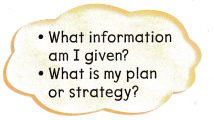Question 1.
There are 10 stickers. 7 stickers are orange. The rest are brown. How many stickers are brown?10 – 7 = ___________
Given,
There are 10 stickers. 7 stickers are orange
10 – 7 = 3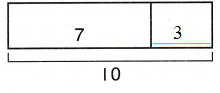Thus 3 stickers are brown.

Question 2.
Some birds were in the tree. 2 birds flew away. Then there were 6 birds. How many birds were in the tree before?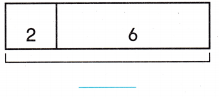________ – 2 = 6
Some birds were in the tree. 2 birds flew away. Then there were 6 birds.
6 + 2 = 8
Thus 8 birds were in the tree before

Question 3.
There were 5 cars. Some cars drove away. Then there was 1 car. How many cars drove away?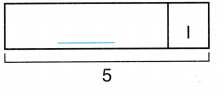5 – ________ = 1
Given,
There were 5 cars.
Some cars drove away.
Then there was 1 car.
5 – 1 = 4
Thus 4 cars drove away.

Math Talk
Mathematical Processes

What does each part of the model show? Explain.

Share and Show

Useto help you. Complete the model and the number sentence.

Question 4.
Some goats were in the field. 3 goats ran away. Then there were 4 goats. How many goats were in the field before?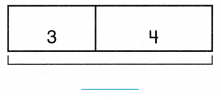_________ – = 4
Given,
Some goats were in the field.
3 goats ran away.
Then there were 4 goats.
3 + 4 = 7
Thus 7 goats were in the field before.

Problem Solving

Question 5.
H.O.T. Write your own story problem using the model.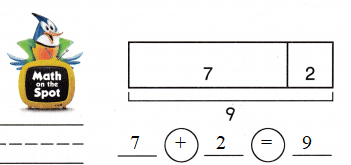Question 6.
H.O.T. Multi-Step Complete the model. Write your own story problem using the model.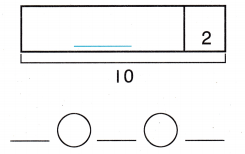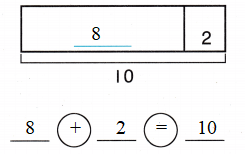Use. Choose the correct answer.

Question 7.
Use Diagrams Peg buys some bananas. Dan eats 3 bananas. Now there are 4 bananas left. How many bananas did Peg buy?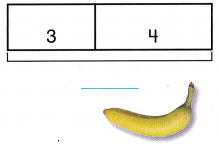(A) 4
(B) 7
(C) 6
Given,
Dan eats 3 bananas. Now there are 4 bananas left.
3 + 4 = 7
Thus the correct answer is option B.

Question 8.
Mari has 11 balloons. 6 balloons are blue. The rest are orange. How many balloons are orange?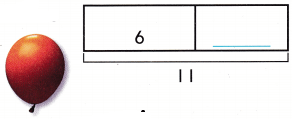(A) 5
(B) 6
(C) 11
Given,
Mari has 11 balloons.
6 balloons are blue. The rest are orange.
6 + 5 = 11
Thus the correct answer is option A.

Question 9.
Marta had 7 grapes. She ate some, Now she has 3. Which number sentence shows how many grapes Marta ate?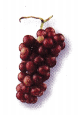(A) 7 – 5 = 2
(B) 7 – 6 = 1
(C) 7 – 4 = 3
Given,
Marta had 7 grapes. She ate some, Now she has 3.
7 – 4 = 3
Thus the correct answer is option C.

Question 10.
Texas Test Prep There are 7 toy rockets. 4 toy rockets are red. The rest are black. How many toy rockets are black?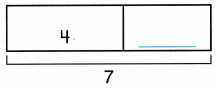(A) 3
(B) 7
(C) 4
Given,
There are 7 toy rockets.
4 toy rockets are red.
The rest are black.
4 + 3 = 7
Thus the correct answer is option A.

TAKE HOME ACTIVITY • Ask your child to describe what the bottom part of a model means when subtracting.

### Texas Go Math Grade 1 Lesson 11.2 Homework and Practice Answer Key

Complete the model and number sentence.

Question 1.
There are 9 cups. 3 cups are white. The rest are red. How many cups are red?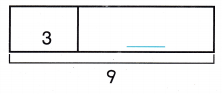9 – 3 = _________
Given,
There are 9 cups. 3 cups are white. The rest are red.
9 – 3 = 6
Thus 6 cups are red.

Question 2.
8 mice were playing. Some mice ran away. Then there were 2 mice. How many mice ran away?8 – 2 = _________
Given,
8 mice were playing. Some mice ran away. Then there were 2 mice.
8 – 2 = 6

Problem Solving

Question 3.
Multi Step Complete the model. Write your own story problem using the model.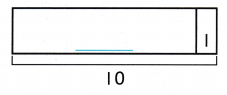9 + 1 = 10

Lesson Check

Question 4.
Tad has 6 shirts. 2 shirts are white. The other shirts are blue. How many shirts are blue?(A) 8
(B) 6
(C) 4
Given,
Tad has 6 shirts. 2 shirts are white. The other shirts are blue.
4 + 2 = 6
Thus the correct answer is option C.

Question 5.
Tom had some pencils. He gave 7 pencils to friends. Now he has 3 pencils. How many pencils did Tom have?(A) 10
(B) 4
(C) 5
Given,
He gave 7 pencils to friends. Now he has 3 pencils.
7 + 3 = 10
Thus the correct answer is option A.

Question 6.
Pat had 9 apples. He used some to make a pie. Now he has 5 apples. Which number sentence shows how many apples Pat used?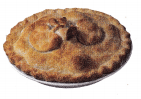(A) 9 – 7 = 2
(B) 9 – 4 = 5
(C) 9 – 6 = 3
Given,
He used some to make a pie.
Now he has 5 apples.
9 – 4 = 5
Thus the correct answer is option B.

Question 7.
11 bears are in the woods. 6 bears are black. The rest are brown. How many bears are brown?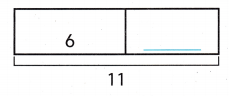(A) 7
(B) 6
(C) 5